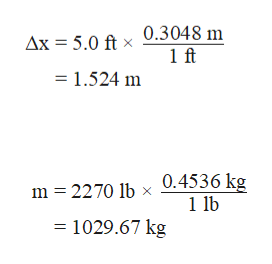# Consider a 2270 lb automobile clocked by law‑enforcement radar at a speed of 85.5 mph (miles per hour). If the position of the car is known to within 5.0 ft at the time of the measurement, what is the uncertainty in the velocity of the car?

Question
778 views

Consider a 2270 lb automobile clocked by law‑enforcement radar at a speed of 85.5 mph (miles per hour). If the position of the car is known to within 5.0 ft at the time of the measurement, what is the uncertainty in the velocity of the car?

check_circle

star
star
star
star
star
1 Rating
Step 1

Refer to the question and calculate the uncertainty in the velocity of the car from the given data.

Uncertainty in the velocity of the car can be calculated by the use of the Heisenberg’s uncertainty principle that is given below.:

Here,

Δx is the uncertainty in the position and Δpx is the uncertainty in the momentum.

h is the Planck’s constant.

Step 2

Uncertainty in the velocity can be calculated as follows:

Step 3

1 feet = 0.3048 meters

Now uncertainty in the position can be calculated as follows:

Since the mass of the ca...help_outlineImage TranscriptioncloseAx = 5.0 ft x 0.3048 m 1 ft = 1.524 m m 2270 lb x 0.4536 kg 1 lb = 1029.67 kg fullscreen

### Want to see the full answer?

See Solution

#### Want to see this answer and more?

Solutions are written by subject experts who are available 24/7. Questions are typically answered within 1 hour.*

See Solution
*Response times may vary by subject and question.
Tagged in

### Atomic structure